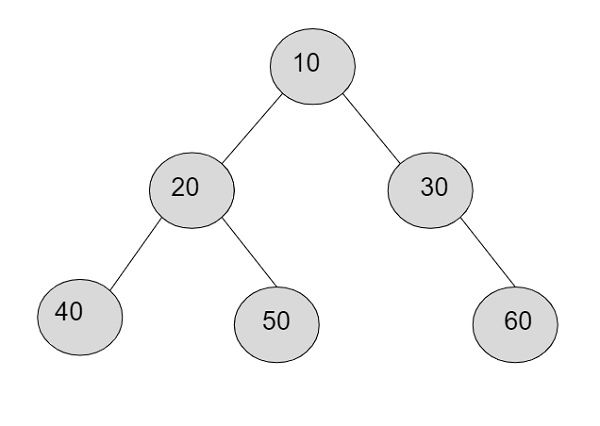# Product of all nodes in a Binary Tree in C++

Given with a binary tree containing nodes and the task is to find the product of all the nodes of a given binary tree.

In a binary tree, there is a root node which is the master node of all the nodes in a tree. A node contains data part, left pointer which will further create left subdirectory and a right pointer which will help in creating right subdirectory. So to traverse the tree, we can take a temporary pointer that will associate with left pointer to traverse left subdirectory or right pointer to traverse the right sub directory.

InputOutput

Nodes are-: 10, 20, 30, 40, 50, 60
Product = 10*20*30*40*50*60 = 72,00,00,000

## Approach

• Input the node data

• Traverse all the nodes starting from the root node and going to either left sub directory or right subdirectory for traversal.

• Store the nodes data and keep multiplying the stored data with the new data

• Print the value of a temporary variable holding the multiplied values.

## Algorithm

Start
Step 1 → create structure of a node
structure node
struct node
int data
Create node *left, *right
End
Step 2 → declare function to insert a node in a tree
node* new_node(int data)
Set node* temp = new node()
Set temp→data = data
Set temp→left = temp→right = NULL
return temp
End
Step 3 → Declare a function to multiply all the nodes
void leaf(node* root, int &product)
IF root = NULL
return 1
End
return (root→data * node_product(root→left) *
node_product(root→right))
Step 4 → In main()
Create node* root = new_node(10)
Set root→left = new_node(20)
Set root→left→left = new_node(30)
Set int product = node_product(root)
Display product
Stop

## Example

Live Demo

#include <iostream>
using namespace std;
//structure of a node
struct node{
int data;
node *left, *right;
};
//function for inserting a new node
node* new_node(int data){
node* temp = new node();
temp→data = data;
temp→left = temp→right = NULL;
return temp;
}
//function for multiplying all the nodes
int node_product(node* root){
if (root == NULL)
return 1;
return (root→data * node_product(root→left) * node_product(root→right));
}
int main(){
node* root = new_node(10);
root→left = new_node(20);
root→right = new_node(30);
root→left→left = new_node(40);
root→left→right = new_node(50);
root→right→left = new_node(60);
int product = node_product(root);
cout << "Product of all the nodes is: "<<product<< endl;
return 0;
}

## Output

If run the above code it will generate the following output −

Product of all the nodes is: 720000000